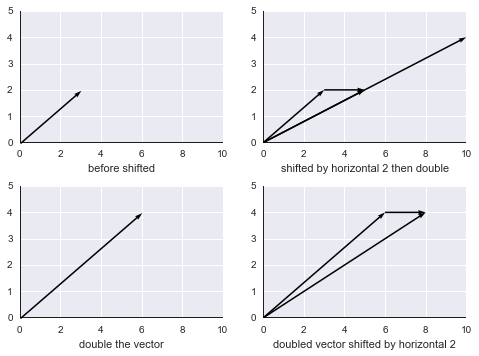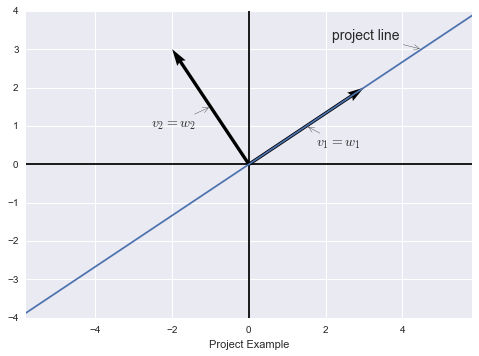# 第三十一讲：线性变换及对应矩阵

$T(v+w)=T(v)+T(w)\\ T(cv)=cT(v)$

“坏”例1，二维空间的平移操作，即平面平移：

%matplotlib inline
import matplotlib.pyplot as plt
import seaborn as sns
import numpy as np

fig = plt.figure()

sp1 = plt.subplot(221)
vectors_1 = np.array([[0,0,3,2],])
X_1, Y_1, U_1, V_1 = zip(*vectors_1)
plt.axhline(y=0, c='black')
plt.axvline(x=0, c='black')
sp1.quiver(X_1, Y_1, U_1, V_1, angles='xy', scale_units='xy', scale=1)
sp1.set_xlim(0, 10)
sp1.set_ylim(0, 5)
sp1.set_xlabel("before shifted")

sp2 = plt.subplot(222)
vector_2 = np.array([[0,0,3,2],
[3,2,2,0],
[0,0,5,2],
[0,0,10,4]])
X_2,Y_2,U_2,V_2 = zip(*vector_2)
plt.axhline(y=0, c='black')
plt.axvline(x=0, c='black')
sp2.quiver(X_2, Y_2, U_2, V_2, angles='xy', scale_units='xy', scale=1)
sp2.set_xlim(0, 10)
sp2.set_ylim(0, 5)
sp2.set_xlabel("shifted by horizontal 2 then double")

sp3 = plt.subplot(223)
vectors_1 = np.array([[0,0,6,4],])
X_1, Y_1, U_1, V_1 = zip(*vectors_1)
plt.axhline(y=0, c='black')
plt.axvline(x=0, c='black')
sp3.quiver(X_1, Y_1, U_1, V_1, angles='xy', scale_units='xy', scale=1)
sp3.set_xlim(0, 10)
sp3.set_ylim(0, 5)
sp3.set_xlabel("double the vector")

sp4 = plt.subplot(224)
vector_2 = np.array([[0,0,6,4],
[6,4,2,0],
[0,0,8,4]])
X_2,Y_2,U_2,V_2 = zip(*vector_2)
plt.axhline(y=0, c='black')
plt.axvline(x=0, c='black')
sp4.quiver(X_2, Y_2, U_2, V_2, angles='xy', scale_units='xy', scale=1)
sp4.set_xlim(0, 10)
sp4.set_ylim(0, 5)
sp4.set_xlabel("doubled vector shifted by horizontal 2")

plt.draw()plt.close(fig)


“坏”例2，求模运算，$$T(v)=\|v\|,\ T:\mathbb{R}^3\to\mathbb{R}^1$$，这显然不是线性变换，比如如果我们将向量翻倍则其模翻倍，但如果我将向量翻倍取负，则其模依然翻倍。所以$$T(-v)\neq -T(v)$$

$v=c_1v_1+c_2v_2+\cdots+c_nv_n\tag{1}$

$T(v)=c_1T(v_1)+c_2T(v_2)+\cdots+c_nT(v_n)\tag{2}$

$$1$$式中，$$c_1,c_2,\cdots,c_n$$就是向量$$v$$在基$$v_1,v_2,\cdots,v_n$$上的坐标，比如分解向量$$v=\begin{bmatrix}3\\2\\4\end{bmatrix}=3\begin{bmatrix}1\\0\\0\end{bmatrix}+2\begin{bmatrix}0\\1\\0\end{bmatrix}+4\begin{bmatrix}0\\0\\1\end{bmatrix}$$，式子将向量$$v$$分解在一组标准正交基$$\begin{bmatrix}1\\0\\0\end{bmatrix},\begin{bmatrix}0\\1\\0\end{bmatrix},\begin{bmatrix}0\\0\\1\end{bmatrix}$$上。当然，我们也可以选用矩阵的特征向量作为基向量，基的选择是多种多样的。

fig = plt.figure()

vectors_1 = np.array([[0, 0, 3, 2],
[0, 0, -2, 3]])
X_1, Y_1, U_1, V_1 = zip(*vectors_1)
plt.axis('equal')
plt.axhline(y=0, c='black')
plt.axvline(x=0, c='black')
plt.quiver(X_1, Y_1, U_1, V_1, angles='xy', scale_units='xy', scale=1)
plt.plot([-6, 12], [-4, 8])
plt.annotate('$v_1=w_1$', xy=(1.5, 1), xytext=(10, -20), textcoords='offset points', size=14, arrowprops=dict(arrowstyle="->"))
plt.annotate('$v_2=w_2$', xy=(-1, 1.5), xytext=(-60, -20), textcoords='offset points', size=14, arrowprops=dict(arrowstyle="->"))
plt.annotate('project line', xy=(4.5, 3), xytext=(-90, 10), textcoords='offset points', size=14, arrowprops=dict(arrowstyle="->"))

ax = plt.gca()
ax.set_xlim(-5, 5)
ax.set_ylim(-4, 4)
ax.set_xlabel("Project Example")

plt.draw()plt.close(fig)


• 确定输入空间的基$$v_1,v_2,\cdots,v_n$$，确定输出空间的基$$w_1,w_2,\cdots,w_m$$
• 计算$$T(v_1)=a_{11}w_1+a_{21}w_2+\cdots+a_{m1}w_m$$，求出的系数$$a_{i1}$$就是矩阵$$A$$的第一列；
• 继续计算$$T(v_2)=a_{12}w_1+a_{22}w_2+\cdots+a_{m2}w_m$$，求出的系数$$a_{i2}$$就是矩阵$$A$$的第二列；
• 以此类推计算剩余向量直到$$v_n$$
• 最终得到矩阵$$A=\left[\begin{array}{c|c|c|c}a_{11}&a_{12}&\cdots&a_{1n}\\a_{21}&a_{22}&\cdots&a_{2n}\\\vdots&\vdots&\ddots&\vdots\\a_{m1}&a_{m2}&\cdots&a_{mn}\\\end{array}\right]$$

• 设输入为$$c_1+c_2x+c_3x^3$$，基为$$1,x,x^2$$
• 则输出为导数：$$c_2+2c_3x$$，基为$$1,x$$

所以我们需要求一个从三维输入空间到二维输出空间的线性变换，目的是求导。求导运算其实是线性变换，因此我们只要知道少量函数的求导法则（如$$\sin x, \cos x, e^x$$），就能求出它们的线性组合的导数。

$$A\begin{bmatrix}c_1\\c_2\\c_3\end{bmatrix}=\begin{bmatrix}c_2\\2c_3\end{bmatrix}$$，从输入输出的空间维数可知，$$A$$是一个$$2\times 3$$矩阵，$$A=\begin{bmatrix}0&1&0\\0&0&2\end{bmatrix}$$